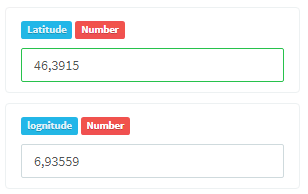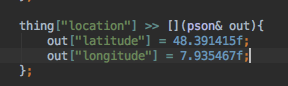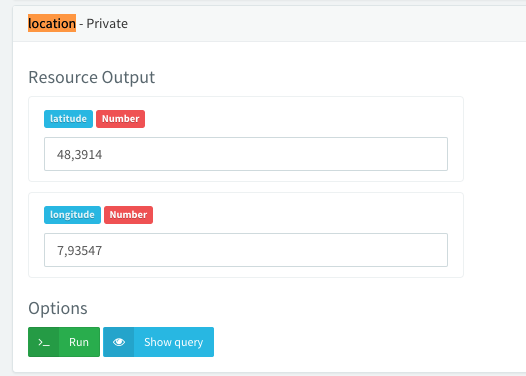# Thinger pson& out cuts numbers behind coma and routd strange

Hi everyone.

I would need your quick help.

I read my GPS Sensor and wanted to mount it to the Thinger Platform.

Here is my Code:

``````        thing["GPSData"] << [](pson& out){
myfile.open("/var/log/thinger.txt", ofstream::out | ofstream::app);
myfile << currentDateTime() << " | Start IP Thing: " << endl;

int fd = openPort("/dev/ttyUSB0");
int i=1;
while (i) {
float lat, lon;
getLocation(fd,&lat,&lon);

int intlat = (int)lat;
int intlon = (int)lon;

if (intlat == 0){
//                    cout << "GPS is not Ready: " << lat << " | " << lon << endl;
}

if (intlat != 0){
cout << "lat: " << lat << endl;
cout << "lon: " << lon << endl;
out["Latitude"] = lat;
out["Lognitude"] = lon;
i=0;
}
}
myfile.close();
};

``````

When I run thinger, the two cout gives me the following output, which is correct:

lat: 48.391415
lon: 7.935467

But on the Thinger Server i got the followingHow can I correct my Code that the Longitude and Latitude becomes equivalent to the Cout.

Many Thanks

Roman

Maybe you can try to convert it to string just to show the values.

Hi ega,

I have successfully changed the above Code

``````double toDouble(float fValue)
{
char czDummy;
printf(czDummy,"%9.5f",fValue);
double dValue = strtod(czDummy,NULL);
return dValue;
}

thing["GPSData"] << [](pson& out){
myfile.open("/var/log/thinger.txt", ofstream::out | ofstream::app);
myfile << currentDateTime() << " | Start IP Thing: " << endl;

int fd = openPort("/dev/ttyUSB0");
int i=1;
while (i) {
float lat, lon;
getLocation(fd,&lat,&lon);

int intlat = (int)lat;
int intlon = (int)lon;

if (intlat == 0){
//                    cout << "GPS is not Ready: " << lat << " | " << lon << endl;
}

if (intlat != 0){
double dlon = lon;
auto strlon = to_string(dlon);
double dlat = lat;
auto strlat = to_string(dlat);
out["Latitude"] = strlat;
out["Lognitude"] = strlon;
i=0;
}
}
myfile.close();
};
``````

Have a nice Day

Roman

I cannot reproduce the issue:

I have tested defining a resource with a fixed location:And it seems to appear correctly in the API explorer:Hello,

Sorry but I see that in the Code you have at latitude less precision (instead of 6 you get 4 and on longitude instead of 6 you have 5. In my point of view the Web api explorer should display the two values with the exact precision of the out[] code

I think you should review the code behind that…

Roman

Hello,

You have just reproduced it…!
See what precision you have behind the coma in your Code and in the Web…
Code = 48.391415
API Explorer= 48.3914 -> Two numbers behind the Coma is cut

Thanks to Fix this Problem

Roman